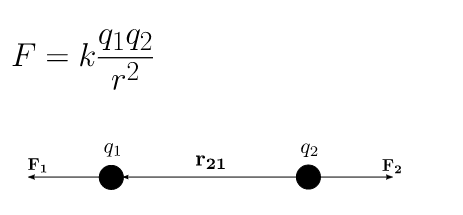Complimentary 1-hour tutoring consultation

MCAT Content / Electrostatics / Coulombs Law

### Coulomb’s Law

Topic: Electrostatics

According to Coulomb’s Law, the force between 2 charges is proportional to both charges and inversely proportional to the distance between the charges.

Electric charge is a property that produces forces that can attract or repel matter. The formula describing the interactions between charges is remarkably similar to that which characterizes the interactions between masses. For electric fields, the force (F) is related to the charges (q1, q2) and the distance (r) between them as:The formula for gravitational force has precisely the same form as Coulomb’s Law, but relates the product of two masses (rather than the charges) and uses a different constant. Both act in a vacuum and are central (depend only on the distance between the forces) and conservative (independent of the path taken). However, it should be noted that when comparing similar terms, charge-based interaction is substantially higher than that based on mass. For example, the electric repulsion between two electrons is about 1042 times stronger than their gravitational attraction.

Coulomb’s constant is ke ≈ 9×10^9 N⋅m2⋅C−2

Practice Questions

MCAT Official Prep (AAMC)

Physics Question Pack Question 44

Physics Question Pack Passage 17 Question 100

Physics Question Pack Passage 17 Question 103

Key Points

• Coulomb’s law quantifies the interaction between 2 stationary charges. It is proportional to both charges and inversely proportional to the distance between the charges.

Key Terms

masses: physical property of matter that depends on size and shape of matter, and is expressed as kilograms by the SI system.

coulomb’s constant is ke ≈ 9×109 N⋅m2⋅C−2

electric charge: a physical property of matter that causes it to experience a force when placed in an electromagnetic field

electric field: a region around a charged particle or object within which a force would be exerted on other charged particles or objects

coulomb’s law: The magnitude of the electrostatic force of attraction or repulsion between two point charges is directly proportional to the product of the magnitudes of charges and inversely proportional to the square of the distance between them

Billing Information